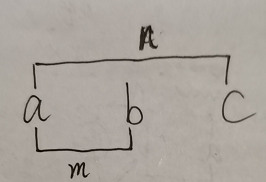• 今天尝试自己编了一个小程序，用来检验两个数大小。下面是程序的部分，有什么不对的欢迎大家指正。 程序部分 #include<stdio.h> #include<Windows.h> #pragma warning(disable:4996) int main() { ...
我的第一个小程序 今天尝试自己编了一个小程序，用来检验两个数的大小。下面是程序的部分，有什么不对的欢迎大家指正。 程序部分 #include<stdio.h> #include<Windows.h>
#pragma warning(disable:4996) int main() { while (1){ int num1 = 0; int num2 = 0; printf(“请输入两个数：”); scanf("%d %d", &num1, &num2); if (num1 > num2){ printf("%d是较大的数\n", num1); } else if (num1 < num2){ printf("%d是较大的数\n", num2); } else if (num1==num2) { printf("%d与%d一样大\n", num1, num2); } } return 0; system(“pause”); }
展开全文• 比较两个数大小 给有需要的朋友，不过我希望朋友们理解后自己写 #include <stdio.h> #include <stdlib.h> int main() { int x,y; printf("请输入两个数进行比较"); scanf("%d%d",&x,&y); ...


比较两个数的大小

给有需要的朋友，不过我希望朋友们理解后自己写
#include <stdio.h>
#include <stdlib.h>

int main()
{
int x,y;
printf("请输入两个数进行比较");
scanf("%d%d",&x,&y);
if(x>y)
printf("由大到小：%d  %d\n",x,y);
else
printf("由大到小：%d  %d\n",y,x);
return 0;
}


展开全文• printf("请输入两个实数：\n"); scanf("%d%d",&a,&b); if(a>b) { printf("%d,%d",b,a); } else printf("%d,%d",a,b); return 0; } //一次比较 ，一次交换，为了实现互换，必须借助于第3个变量。 ...
//两次比较，比较简单。
/*#include <stdio.h>
int main(void)
{
int a,b;
printf("请输入两个实数：\n");
scanf("%d%d",&a,&b);
if(a>b)
{
printf("%d,%d",b,a);
}
else
printf("%d,%d",a,b);
return 0;
}

//一次比较 ，一次交换，为了实现互换，必须借助于第3个变量。

#include<stdio.h>
int main (void)
{
int a,b,t;
printf("请输入两个实数：\n");
scanf("%d%d",&a,&b);
if(a>b)
{
t=a;
a=b;
b=t;
}
printf("%d,%d",a,b);
return 0;
}

//三个数比较大小

#include<stdio.h>
int main (void)
{
int a,b,c,t;
printf("请输入三个实数：\n");
scanf("%d%d%d",&a,&b,&c);
if(a>b)
{
t=a;
a=b;
b=t;
}
if(a>c)
{
t=a;
a=c;
c=t;
}
if(b>c)
{
t=b;
b=c;
c=t;
}
printf("%d,%d,%d",a,b,c);
return 0;

}

展开全文• 1）唯一的三目运算符(条件运算符)可以用来获得两个数中最大或最小的那个。形式上更加简洁。 2）条件表达式的本质就是if-else。冒号：后的值就是else语句。 举例1：条件运算符的嵌套： c = ...
方法1：使用if-else比较
if(a>=b)
c = a;
else
c = b;
方法2：使用条件运算符比较
c = a>=b?a:b;
总结：
1）唯一的三目运算符(条件运算符)可以用来获得两个数中最大或最小的那个数。形式上更加简洁。
2）条件表达式的本质就是if-else。冒号：后的值就是else语句。
举例1：条件运算符的嵌套：
c = a>b?2:a==b?1:0;
当a>b时，c = 2;
当a==b时，c = 1;
当a<b时，c=0;
if(a>b)
c = 2;
else if(a == b) //此处else相当于第一个冒号
c = 1;
else        //此处else相当于第二个冒号
c = 0;
举例2-1：求3个数中最大的数
a,b,c三个数，求最大值
if(a>=b)//比较a和b,最大值赋给m
m = a;
else
m = b;

if(a>=c)//比较a和c,最大值赋给n
n = a;
else
n = c;

if(m>=n)//比较m和n,获得最大值
max = m;
else
max = n;
等价于以下条件表达式：
m = a>=b?a:b;
n = a>=c?a:c;
max = m>=n?m:n;
又可以写成
举例2-2：求3个数中最大的数
if(a>=b)//比较a和b,最大值赋给m
m = a;
else
m = b;

if(a>=c)//比较a和c,最大值赋给n
n = a;
else
n = c;

if(b>=c)//比较b和c,获得最大值
max = m;
else
max = n;
等价于条件表达式
max = b>=c?a>=b?a:b:a>=c?a:c;
//max = b>=c?(a>=b?a:b):(a>=c?a:c);


分析：显示2-2方法更简洁，思路分析：
a与b比较：m为二者最大值；
a与c比较：n为二者最大值；
b与c比较：若b>=c,则m为最大值（如m=a,则a>=b>=c;如m=b，则b>=a，且b>=c）;
若b<c,则n为最大值（如n=a,则，b<c,c<=a,即b<c<=a;如n=c,则a<c且b<c）。示意图如下：其实，2-2的程序写成如下更贴切：
if(b>=c)        //以任意两值比较，
{
if(a>=b)    //第3个值与最大值b进行比较 //if-else对应第一个括号的内容，即冒
max = a;    //号前的内容
else
max = b;
}else{            //b<c的情况
if(a>=c)      //第3个值与最大值c进行比较//if-else对应第二个括号中的内容，即
max = a;  //冒号后的内容
else
max = c;
}


总结：三目表达式？：的理解
？前的内容是if中的内容；
：代表else
：前的内容是if语句；
：后的内容是else语句。
展开全文• ## C语言比较两数大小

千次阅读 2017-10-15 20:33:20
#include #include /* run this program using the console pauser or add your own getch, system("pause") or input loop */ int main(int argc, char *argv[]) { ... printf("请输入比较大小两个数：");
•新手
• 定义一个数组，使用for循环来存放输入的十个数字，再使用一个for循环来进行两个数字的大小比较，将较大值放入到max里边，再进行和下一个数值的大小比较，最后输出。 #include<stdio.h> #include<stdlib.h&...算法
• //strcmp (参数1类型为：char *，参数2类型为：char *），用于比较参数1，参数2两个字符串的值大小，返回一个int类型的。如果第一个参数大于第二个参数返回1，相等返回0，小于返回-1，头文件为#include<string....
• } //比较大小的max函数 int max(int x, int y) {//定义max函数，函数值为整型，形式参数x,y为整型 int z;//max函数中的声明部分,定义本函数中用到的变量z为整数 if (x > y)z = x; else z = y; getchar(); return(z)...
• 作用是返回两个浮点参数中较大的一个 #include <stdio.h> #include <math.h> int main(void) { printf("fmax(2,1) = %f\n", fmax(2,1)); printf("fmax(-Inf,0) = %f\n", fmax(-INFINITY,0)); ...
• 比较两数大小 1.利用if else输出较大值 #include<stdio.h> int main() { int a=0,b=0 ; scanf("%d%d", &a, &b); if (a>b) printf("%d", a); else if (a<b) printf("%d", b); ...
• 目录 一、先写好框架 二、然后定义我们需要的变量...今天我们要写的是用调用函数的方法来 比较两个数字的大小 我们先看看程序的运行效果 一、先写好框架 #include<stdio.h> void main() { } 二...函数调用
• 题目要求：输入两个实数，...2.两个数比较大小可以用if条件语句 3.比较大小后把大的存在max变量中 最后输出max就OK啦 代码如下 #include "stdio.h" void main() { int a,b,max; scanf("%d %d",&a,&b); ...
• 唯一的三目运算符(条件运算符)可以用来获得两个数中最大或最小的那个。形式上更加简洁。 方式三 函数方法也可搞定这一问题，先创建一个Max函数，定义如下 再添加主函数main，对调用Max函数进行声明 常用的三种...
• 供大家参考 #include<stdio.h> int mymax(int a,int b) { if(a>b) return a; else return b; } int main() { int x,y,z; printf("input two number:\n"); scanf("%d%d",&......算法
• ## C语言比较三个整数大小

万次阅读 多人点赞 2018-03-09 13:34:22
个数大小：#include &lt;stdio.h&gt;int main(){ int a,b,c; scanf("%d %d %d", &amp;a, &amp;b, &amp;c); int max = 0; if ( a&gt;b ) { if ( a&gt;c ) { max = a; ...C
• gcc #include <stdio.h> int main() { double a,b; a = 2.5; b = 1.9; // printf("input a b\n"); // scanf("%lf %lf",&a,&b); if (a > b) printf("%lf > %lf",a,b);... %lf",a
• ## 三个数比较大小 — C语言经典题目

万次阅读 多人点赞 2017-03-29 20:57:40
以上三种方法均可以比较出三个数的最大值，区别在于例子中的方法是使用的赋值语句，而替代方法1是运用的标准条件运算，后面的替代方法2则是以赋值语句为主的重（条件运算的）嵌套。 补充：替代方法2不能写成max =...
• #include <stdio.h.> #include <windows.h> #pragma warning(disable:4996) int MYMax(int _x, int _y) { if(_x >_y?_x:_y) { return _x; } else{ return _y; } } int main() ...int y = 0...
• 从键盘输入两个数，通过代码判断大小输出较大值。 代码 #include<stdio.h> int main() { int a, b; printf(“输入两个数：”); scanf("%d %d", &a, &b); if (a > b) { printf(“较大值是：%d\n”, ...
• Code: #include void main() { int *p1,*p2,*p,a,b; scanf("%d,%d",&a,&b); p1 = &a; p2 = &b; if (a>b); if(a语言 c
• main() {  int a,i,j,s;  for(i=0;i  scanf("%d",&a[i]); /*输入四个数*/    for(i=0;i  for(j=i+1;j  if(a[i]>a[j]){  s=a[i];iOS ios开发
• //指针变量交换两个数的值，函数内部要交换两个数的值要通过指针交换 { int t = *x; *x = *y; *y = t; } 2.异或法 根据将某个与另一个连续与或两次，可得到本身。将两个数进行与或运算，再分别与...swap函数 异或法
• 如何用传址的方式交换两个数大小 首先先看第一个程序，用函数的调用来解决看似正确实则运行出来的结果是错误的 #define _CRT_SECURE_NO_WARNINGS 1 #include #include #include int JH(int x, int y); int main() ...
•  先比较两个数大小，然后找出较小t，最后判断t为何值时两个数都能整除，此方法效率较低。代码如下：#include int main() {  int num1,num2,temp,i;  scanf("%d%d",&num1,&num2);  if(num1>num2)  {
• #define _CRT_SECURE_NO_WARNINGS 1 #include<stdio.h> #include<stdlib.h> int main() { int num1 = 0; int num2 = 0;...printf(“请输入两个数:”); scanf("%d %d ", &num1, &...
• //输入两个数输出最大值 #include<stdio.h> intmain(intargc,constchar*argv[]){ inta,b,tmp; printf("请输入两个数字:\n"); scanf("%d%d",&a,&b); if(b>a){ ...
• #include <stdio.h> int max(int x,int y) { if(x>=y) return x; else return y;...printf("请输入2数字:\n"); scanf("%d%d",&a,&b); printf("最大值为:%d\n",max(a,b)); } ...
• ## c语言入门：比较三个数的大小

万次阅读 多人点赞 2017-10-30 23:13:25
这是最容易理解的方法在之前判断两个数大小的基础上，在if语句中嵌套一个if语句，经过多次判断得出最大。 这样可以再引用函数中将引用的函数变为max（a,b,c）或者在比较两个数的函数在主程序里变为max=ma...

# c语言比较两个数的大小c语言 订阅